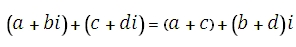# SQL Server IMSUM function

Updated: 27 August 2010

Use IMSUM to return the sum of a dataset of complex numbers in x + yi or x + yj text format. The sum of 2 complex numbers is computed as:Syntax
SELECT [wctEngineering].[wct].[IMSUM] (
<@IMValues_Tablename, nvarchar(4000),>
,<@ColumnName, nvarchar(4000),>
,<@GroupedColumnName, nvarchar(4000),>
,<@GroupedColumnValue, sql_variant,>)
Arguments
@IMValues_Tablename
the name, as text, of the table or view that contains the complex numbers to be used in the IMSUM calculation.
@ColumnName
the name, as text, of the column in the table or view specified by @IMValues_Tablename that contains the complex numbers to be used in the IMSUM calculation.
@GroupedColumnName
the name, as text, of the column in the table or view specified by @IMValues_Tablename which will be used for grouping the results.
@GroupedColumnValue
the column value to do the grouping on.
Return Types
varchar(4000)
Remarks
·         Use COMPLEX to convert real and imaginary coefficients into a complex number.
·         For more complex queries consider using the IMSUM_q function.
Examples

create
table #s1 (inumber varchar(50))
insert into #s1 values ('2+i')
insert into #s1 values ('2-i')
insert into #s1 values ('3+2i')
insert into #s1 values ('3-2i')

select
wct.imsum('#s1','inumber','',NULL)

This produces the following result
-------------------------
10

(1 row(s) affected)

select
wct.IMADD(wct.IMADD('2+i','2-i'), wct.IMADD('3+2i','3-2i'))

This produces the following result
------------------------
10

(1 row(s) affected)

### PricingCopyright 2008-2019 Westclintech LLC         Privacy Policy        Terms of Service